## Circular Functions

The functions describing the horizontal and vertical positions of a point on a Circle as a function of Angle (Cosine and Sine) and those functions derived from them: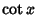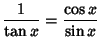(1)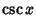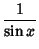(2)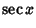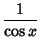(3)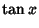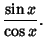(4)

The study of circular functions is called Trigonometry.

See also Cosecant, Cosine, Cotangent, Elliptic Function, Generalized Hyperbolic Functions, Hyperbolic Functions, Secant, Sine, Tangent, Trigonometry

References

Abramowitz, M. and Stegun, C. A. (Eds.). Circular Functions.'' §4.3 in Handbook of Mathematical Functions with Formulas, Graphs, and Mathematical Tables, 9th printing. New York: Dover, pp. 71-79, 1972.